In :
import pymc
import daft
import csv
from scipy.sparse import coo_matrix
from itertools import product, izip


# Welcome¶

Bayesian networks are a method for building your own machine learning models.

• With Bayesian networks, we model our data problem as a causal network, or a story involving hidden and observed variables.
• We come up with a story that we think explains our data.
• We use Bayes's rule to find posterior probability distributions of the hidden variables given our observed variables (data).
• We use the full posterior distributions for our next action (decision, recommendation, prediction).
• Confidence estimates.
• Flexibility. We can change the story and re-train the model.
• Classical machine learning methods are inflexible: Code and theory only works for its specific problem.
• Your real world data probably has some important Difficult to adapt to your particular problem, which may not have been studied by researchers.
• Slow (unless you do a lot of math)
• You have to think.
• For many of your data problems, you may want or need to fit a custom machine learning model.

# Bayesian Statistics¶

We have some data $X$. How did it get there?

We don't know. There is no one right answer, only answers that are more or less supported by the evidence.

A textbook example: Somebody tests HIV positive.

Story:

• Grab a person off the street in New York. (0.03% of NY is diagnosed with HIV)
• Give them an HIV test.
• For HIV positive patients, test returns positive 99% of the time. (True positive rate.)
• For HIV negative patients, test returns negative 0.1% of the time. (False positive rate.)

Does the person have HIV? We don't know. But we can compute probabilities, given this story, using Bayes' rule.

Let $+$ denote the event the 'test positive', $-$ denote the event 'test negative', and $H$ denote the event 'HIV positive'. Then using Bayes's rule, $$\begin{eqnarray*} p(H|+) & = & \frac{p(+|H)p(H)}{p(+)} \\\\ & = & \frac{p(+|H)P(H)}{p(+|H)p(H)+p(+|\overline{H})p(\overline{H})} \\\\ & = & \frac{(0.99)(0.0003)}{(0.99)(0.0003)+(0.001)(0.9997)} \\\\ & \approx & 0.229 \end{eqnarray*}$$

## Bayesian Statistics, More Formally¶

To do Bayesian statistics, we need

• A probability model, a story of how the data came to be.
• Hidden variables $\theta$, about which which we want to infer probabilities.
• Observed variables $X$.
• A prior on our hidden variables $p(\theta)$: What do we believe before seeing any data?
• A likelihood $p(X | \theta)$ relating hidden variables to observed variables

Then Bayes' rule ties them together:

$$\begin{eqnarray*} p(\theta|X) & = & \frac{p(X|\theta)p(\theta)}{p(X)} \\\\ & = & \frac{p(X|\theta)P(\theta)}{\int p(X|\theta)p(\theta) d\theta} \\\\ \end{eqnarray*}$$

Bayesian inference is

• Useful: hidden variables
• Hard: the denominator is high-dimensional integral. You can't just use Simpson's rule; it will take exponential time.
• We use Markov Chain Monte Carlo (MCMC) with the PyMC package.

# Movie Recommendations¶

We have $N$ users and $M$ items. Given a small samples of ratings $r_{ij}$, predict $\hat{r}_{i'j'}$ for pairs $(i', j')$ we don't observe.

Our toy dataset: http://mlcomp.org/datasets/736, 190 training ratings (81 test), $N = 6, M = 91$.

In :
def loadData(filename):
users = data[:,0].astype(int)
items = data[:,1].astype(int)
ratings = data[:,2]

nExamples = len(users)

return (nExamples, users, items, ratings)

TRAIN_FILE = '736/train'
TEST_FILE  = '736/test'

(nTrain, users, items, ratings) = loadData(TRAIN_FILE)
(nTest,  testUsers, testItems, testRatings) = loadData(TEST_FILE)

N = np.max(users)
M = np.max(items)


Always look at our data!

In :
plt.figure()
plt.hist(ratings)
plt.figure()
plt.hist(testRatings)

Out:
(array([ 47.,  18.,   9.,   2.,   0.,   3.,   1.,   0.,   0.,   1.]),
array([ 0.75432862,  1.2940267 ,  1.83372478,  2.37342286,  2.91312094,
3.45281902,  3.9925171 ,  4.53221518,  5.07191326,  5.61161134,
6.15130942]),
<a list of 10 Patch objects>)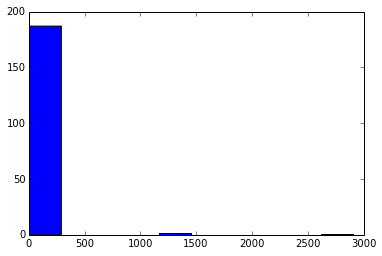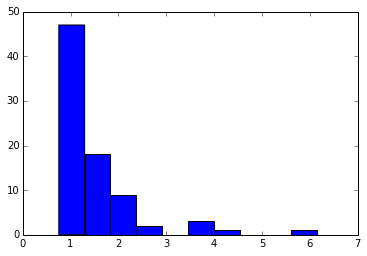From the test set, it appears like we have 1-6 stars, but something weird is happening in the training set! The histogram says there are outliers, so let's take a look:

In :
outlierIdxs = ratings > 6
print np.flatnonzero(outlierIdxs)
print ratings[outlierIdxs]

[ 83 163 165 167 168]
[    9.54361436  1379.98131862     8.76865101  2907.19215935  1380.47446215]


I delete the outliers from my data file; much better. Always make a new copy; do not overwrite your original data!

In :
CLEAN_TRAIN_FILE = '736/trainClean'
(nTrain, users, items, ratings) = loadData(CLEAN_TRAIN_FILE)

plt.hist(ratings)

Out:
(array([ 95.,  43.,  17.,   5.,   5.,   3.,  13.,   1.,   1.,   2.]),
array([ 0.75722523,  1.23237711,  1.70752898,  2.18268086,  2.65783273,
3.13298461,  3.60813648,  4.08328836,  4.55844023,  5.03359211,
5.50874398]),
<a list of 10 Patch objects>)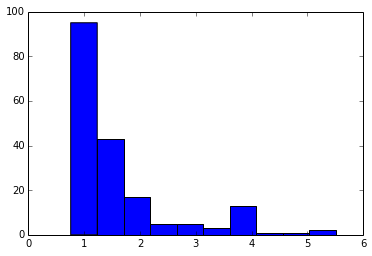Now that we've taken a look at our data, let's tell a story.

The classic matrix factorization method for recommendations represents users and items as $d$-dimensional vectors.

• Each dimension represents some abstract "taste": horror? chick flick? comedy?
• A rating $r_{ij}$ is just the dot product of the respective user and item vectors: $\mathbf{u}_i ^\top \mathbf{v}_j$.

In Bayesian land,

• For user $i = 1, \ldots, N$, draw $\mathbf{u}_i \sim \mathcal{N}(0, \tau_0 I_d)$
• For item $j = 1, \ldots, M$ draw $\mathbf{v}_j \sim \mathcal{N}(0, \tau_0 I_d)$
• For $i = 1, \ldots, N$ and $j = 1, \ldots, M$,

• Compute $z_{ij} = \mathbf{u}_i ^\top \mathbf{v}_j$
• Draw $r_{ij} \sim \mathcal{LN}(z_{ij}, \tau_1)$.

The Bayesian network looks like

In :
from matplotlib import rc
rc("font", family="serif", size=36)
rc("text", usetex=True)

pgm = daft.PGM([5, 4], grid_unit=5, node_unit=3)
pgm.add_node(daft.Node("tau0", r"$\tau_0$",    2, 3.5, fixed=True))
pgm.add_node(daft.Node("u", r"$\mathbf{u}_i$", 1, 2.5))
pgm.add_node(daft.Node("v", r"$\mathbf{v}_j$", 3, 2.5))

pgm.add_node(daft.Node("z", r"$z_{ij}$", 2, 2.5))
pgm.add_node(daft.Node("r", r"$r_{ij}$", 2, 1.5, observed=True))

pgm.add_node(daft.Node("tau1", r"$\tau_1", 0.25, 1.5, fixed=True)) pgm.add_edge("tau0", "u") pgm.add_edge("tau0", "v") pgm.add_edge("u", "z") pgm.add_edge("v", "z") pgm.add_edge("z", "r") pgm.add_edge("tau1", "r") # [start_x, start_y, xlen, ylen] pgm.add_plate(daft.Plate([0.5, 0.5, 2, 2.5], label=r"$i = 1, \ldots, N$")) pgm.add_plate(daft.Plate([1.5, 0.25, 2, 2.7], shift=0.5, label=r"$j = 1, \ldots, M$")) pgm.render()  Out: <matplotlib.axes.Axes at 0x111c94f10>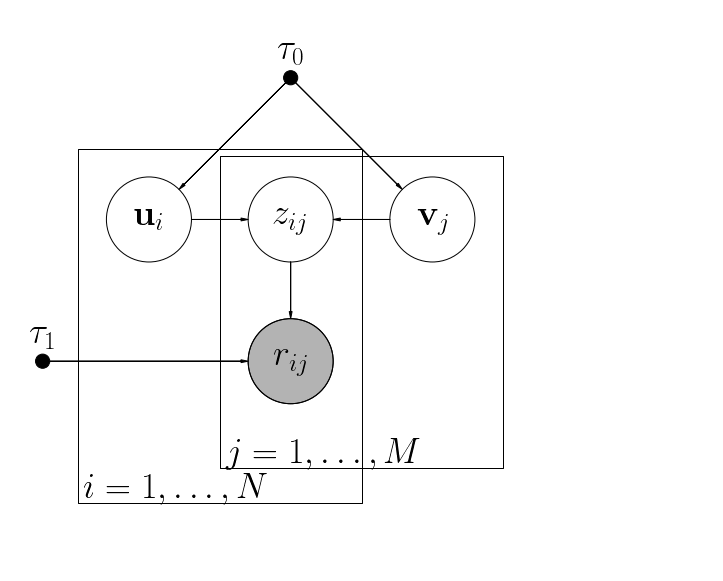Finding hyperparmeters is the unprincipled black magic of machine learning. I basically tried some numbers until they worked. In : D = 10 tau0 = 5 tau1 = 5  In : u = pymc.Normal('u', 0, tau0, size=(D, N)) v = pymc.Normal('v', 0, tau0, size=(D, M)) z = pymc.Lambda('z', lambda u=u, v=v: np.dot(u.T, v)) r = np.empty(nTrain, dtype=object) for n, (i, j) in enumerate(izip(users, items)): r[n] = pymc.Lognormal('x_%d_%d' % (i, j), z[i-1, j-1], # mean tau1, # precision (confidence) observed=True, value=ratings[n]) model = pymc.Model([u, v, z, r])  # Posterior Inference and Markov Chain Monte Carlo¶ In : # There was a bug in PyMC; this is just to hack around code. if 3 in model.__dict__: del model.__dict__ pymc.MAP(model).fit() mcmc = pymc.MCMC(model) mcmc.sample(10000)  [****************100%******************] 10000 of 10000 complete  Joint probability: $$p\left(\left\{ r_{ij},z_{ij}\right\} _{ij\in I}\left\{ \mathbf{u}_{i}\right\} _{i=1}^{N},\left\{ \mathbf{v}\right\} _{j=1}^{M},\tau_{0},\tau_{1}\right)=\prod_{ij\in I}\mathcal{LN}(r_{ij}|\mathbf{u}_{i}^{\top}\mathbf{v}_{i},\tau_{1})\prod_{i=1}^{N}\mathcal{N}(\mathbf{u}_{i},\tau_{0}I_{d})\prod_{j=1}^{M}\mathcal{N}(\mathbf{v}_{j},\tau_{0}I_{d})$$ Posterior probabilities: $$p\left(\left\{ z_{ij}\right\} _{ij\in I}\left\{ \mathbf{u}_{i}\right\} _{i=1}^{N},\left\{ \mathbf{v}\right\} _{j=1}^{M},\tau_{0},\tau_{1}\vert\left\{ r_{ij}\right\} _{ij\in I}\right)=\frac{\prod_{ij\in I}\mathcal{LN}(r_{ij}|\mathbf{u}_{i}^{\top}\mathbf{v}_{i},\tau_{1})\prod_{i=1}^{N}\mathcal{N}(\mathbf{u}_{i},\tau_{0}I_{d})\prod_{j=1}^{M}\mathcal{N}(\mathbf{v}_{j},\tau_{0}I_{d})}{\int\prod_{ij\in I}\mathcal{LN}(r_{ij}|\mathbf{u}_{i}^{\top}\mathbf{v}_{i},\tau_{1})\prod_{i=1}^{N}\mathcal{N}(\mathbf{u}_{i},\tau_{0}I_{d})\prod_{j=1}^{M}\mathcal{N}(\mathbf{v}_{j},\tau_{0}I_{d})d\mathbf{z}d\mathbf{u}d\mathbf{v}}$$ Posterior probabilities are the cornerstone of Bayesian statistics. They give us information like • Predicted ratings,$r_{ij}$• Error bars on predictions,$\sqrt{\mathbf{V}[r_{ij}]}$• Shape of latent representations of movies and users,$p(\mathbf{u}_i | \ldots)$,$p(\mathbf{v}_i | \ldots)$. Markov chain Monte Carlo: (MCMC) Do not evaluate the posterior explicitly. Construct a Markov chain whose invariant distribution is the posterior. In fact, with MCMC we never know how to write down the posterior. Eventually, our Markov chain generates samples from it. • Markov chain: a process whose future depends only on the present, not on its own history • Computationally easy to simulate. • Analytically, rich theory, which justifies the method. Gibbs sampling: The easiest MCMC method. • Let$x_1, \ldots, x_n$be random variables, and$\mathbf{y}$be observed. • For$t = 1, 2, \ldots$: • For$i = 1, \ldots, n$: • Sample$p(x_i | x_1, \ldots, x_{i-1}, x_{i+1}, x_i, \mathbf{y})$Properties: • Each inner loop generates a vector$\mathbf{x}^{(t)}_{1:n}$. These vectors form a Markov chain. • At the invariant distribution (when you run then chain for "long enough"), the Markov chain generates samples from the conditional$p(\mathbf{x} | \mathbf{y})$. • For any subset of variables$x_i, x_j, x_k$, keeping just those variables from the Markov chain is a sample from the marginal distribution$p(x_i, x_j, x_k | \mathbf{y})$. ## Posterior Analysis¶ In : zsamples = mcmc.trace('z')[5000:] zmean = np.mean(zsamples, 0) meanExpZ = np.mean(np.exp(zsamples), 0)  We learned a distribution over all log-ratings$z_{ij}$. Let's take a peek at the first rating. The shape of the histogram captures our knowledge, including knowledge of uncertainty. In : plt.hist(np.exp(zsamples[:,1,1])) iFirst, jFirst, rFirst = users, items, ratings print meanExpZ[iFirst,jFirst] print rFirst  1.36496704562 1.5098059294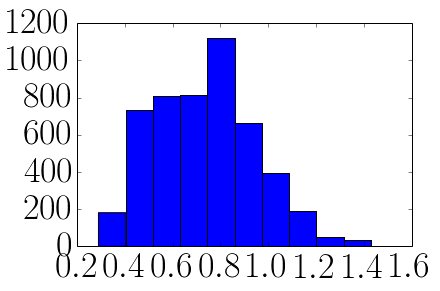## Accuracy Validation¶ Let's output our predicted ratings as the mean rating. Let$I$be the set of item-user pairs rated, with true ratings$r_{ij}$. Recall$z_{ui}\$ is the logarithm of the rating. The standard error metric is root-mean squared error (RMSE):

$$\text{RMSE}(I) = \sqrt{\frac{1}{|I|}\sum_{(i,j)\in I}\left(\exp z_{ij}-r_{ij}\right)^{2}}$$

Let us calculate the RMSE for both training and test sets. In general, if the RMSE is way better for training set, then we've overfit.

In :
#trainRMSE = np.sqrt(np.mean((np.exp(rmean[users-1, items-1]) - ratings) ** 2))
#testRMSE  = np.sqrt(np.mean((np.exp(rmean[testUsers-1, testItems-1]) - testRatings) ** 2))
trainRMSE = np.sqrt(np.mean( (meanExpZ[users-1, items-1] - ratings) ** 2))
testRMSE  = np.sqrt(np.mean( (meanExpZ[testUsers-1, testItems-1] - testRatings) ** 2))

print trainRMSE
print testRMSE

0.686684550069
0.876480474553


## The Competitor, Nonnegative Matrix Factorization¶

Again, I jiggled the sparsity (beta0) knob; these were the best results I got.

Note exactly a fair comparison, since the NMF code is far better vectorized.

In :
R = coo_matrix((ratings, (users - 1, items - 1))).todense()
W = skm.fit_transform(R.T)

reconstruct = np.dot(skm.components_.T, W.T)

nmfTrainRMSE  = np.sqrt(np.mean( (reconstruct[users-1, items-1] - ratings) ** 2))
nmfTestRMSE   = np.sqrt(np.mean( (reconstruct[testUsers-1, testItems-1] - testRatings) ** 2))

print nmfTrainRMSE
print nmfTestRMSE

#print skm.reconstruction_err_
#pint np.linalg.norm(reconstruct - R)

0.907722163866
1.39713801489

In [ ]: